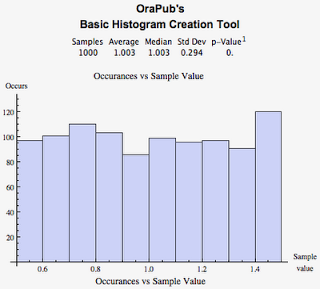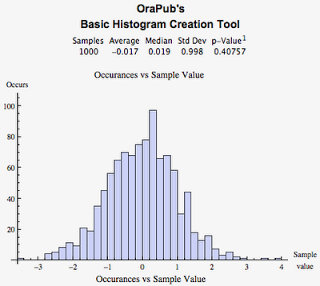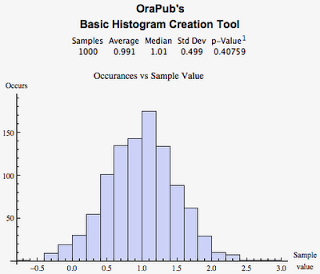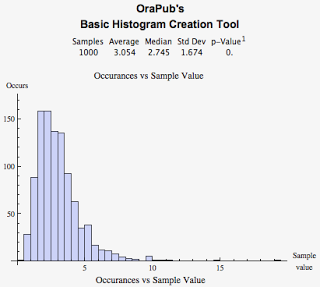## Creating Realistic User Think Times For Research Projects

Posted on 08-May-2012 by Craig Shallahamer, craig@orapub.com

### Creating sensible think times is kind of tricky

My job requires I spend a tremendous amount of time doing Oracle Database performance analysis research. Over the years I have developed a list of things I do to help ensure my research is up to the level I need for a specific experiment. One of the more interesting things on my list is creating a more realistic workload by using sleep times (i.e., think times) that are not constant. When sleep times are the same the system behaves unrealistically constant. On real systems, even a CPU bottlenecked system exhibits an ebb and flow.

Here's an example of what I'm referring to. Let's say we need to create a logical IO (buffer gets) intensive workload. One way to do this is:

• Create a table with 1M rows.
• Create a big enough buffer cache to ensure all the rows will always be cached.
• Create a query that SELECTs from this table.
• Vary the workload intensity by either altering the query itself, increasing the number of sessions executing the query, or by decreasing the "think time" between queries.

All OLTP systems contain think time because there are real users pushing work into the system...and they have to occasionally think. (key word: occasionally) If we want a more realistic behaving system, we need think times to be more than just a constant value.

How you can create more realistic think times is what this blog is all about.

### The trick is, how do we model this think time?

You can download all the plsql mentioned below in this single text file HERE. If you need a quick refresher regarding histograms, read the first part of this blog post.

Constant think time.

An easy option is to simply use a constant time by using dbms_lock.sleep(x) or creating the values using dbms_random.value(x,x). The problem with this, is the resulting workload is not very realistic because users don't exhibit constant think times.

When you observe the load using a tool like vmstat, while there can be utilization ups and downs, you'll notice the utilization will stay unrealistically constant...not very realistic as real systems ebb and flow. Including multiple SQL statements can make for a more variable utilization, but it will likely be very erratic and not the smooth ebb and flow that tends to occur in a real system. Plus the ebb and flow is occurring because the workload changes, but real systems also ebb and flow because user think times are not constant, plus a variety of other reasons.

A more formal way to describe this constant type of think time is each sample think time is pulled from a uniform distribution with the same minimum and maximum values. Here's the plsql to create the sample data:

``````--
-- Code for UNIFORM sample values with min and max of 1.0.
--
declare
max_samples  number := 1000;
sample_value number;
i            number;
begin
dbms_random.seed(13);
for i in 1..max_samples
loop
sample_value := dbms_random.value(1.0, 1.0) ;
dbms_output.put_line(round(sample_value,4));
end loop;
end;
/ ``````

A histogram was not created because all 1000 samples are exactly the same. Picture the Washington Monument and you have a pretty good picture of what it will look like.

Uniform think time.

The next step for many of us is to provide a range of think times. We think, "Uses will think between 0.5 and 1.5 seconds." This can be implemented simply by using dbms_random.value(0.50, 1.50). We can create a uniform distribution samples between 0.50 and 1.50 seconds using the code below.

``````--
-- Code for UNIFORM sample values with min of 0.50 and max of 1.5.
--
declare
max_samples  number := 1000;
sample_value number;
i            number;
begin
dbms_random.seed(13);
for i in 1..max_samples
loop
sample_value := dbms_random.value(0.50, 1.5) ;
dbms_output.put_line(round(sample_value,4));
end loop;
end;
/ ``````Looking at the histogram above, is this what you expected? Probably not. Surely, this can't be production system realistic. While observing the OS utilization will not be as constant as the "constant think time," the ebb and flow is still far from real and will likely be spiky; abrupt increases and decreases.

Normal think time.

Referring to the previous histogram above, I suspect most readers were expecting to see what is called "normal think time." The rational follows this line of thinking. "Users will think an average of one second, sometimes more and sometimes less." The hidden assumption is the more and less likelihood are the same.

Oracle provides the dbms_random.normal function that returns samples with an average of 0 and standard deviation of 1. This is called a standard normal distribution. The code and histogram are shown directly below.

``````-- Code for NORMAL sample values with average of 0.0 and stdev of 1.0.
--
declare
max_samples  number := 1000;
sample_value number;
i            number;
begin
dbms_random.seed(13);
for i in 1..max_samples
loop
sample_value := dbms_random.normal;
dbms_output.put_line(round(sample_value,4)||',');
end loop;
end;
/
``````Important: But this is not what we want! We need a normal distribution with an average of 1.0 and a standard deviation of 0.5. We can easily increase the average by either adding or subtracting from the resulting sample value. But what about altering the dispersion, that is, the variance and standard deviation? It's very simple using Mathematica or R because one of the inputs for the normal distribution function is the standard deviation. Not so with dbms_random.

But with dbms_random, it's not that simple. In fact, altering the dispersion, that is, the variance and standard deviation is not obvious. Surprisingly, I could find no references searching using Google!

So I started digging... and figure it out. Not being a statistician, for me this was a discovery. I recalled that we can standardize values, which results in the infamous z value using the following formula:

z = ( x - m ) / s

Where:

z is the standardized sample value

x is the unstandardized sample value

m is the sample set average

s is the sample set standard deviation

In our situation, we are trying to determine the un-standardized value (x) while dbms_random.normal provides us with a standardized value (z) with a default average (m) of zero, and a default standard deviation (s) of 1. So I figured I would simply set z, m, and s and then solve for x... the unstandardized value!

With a little algebra, solving for x becomes:

x = z * s + m

This is coded in the plsql below followed by the histogram.

``````declare
max_samples  number := 1000;
sample_value number;
i            number;
z            number;          -- standardized value
m            number  := 1.0 ; -- average
s            number  := 0.5 ; -- standard deviation
x            number;          -- un-standardized value
begin
dbms_random.seed(13);
for i in 1..max_samples
loop
z := dbms_random.normal;
x := z * s + m ;
dbms_output.put_line(round(x,4)||',');
end loop;
end;
/ ``````And it worked! The figure above displays an average of 0.991 and a standard deviation of 0.499! I tried the above code snippet with a variety of averages and standard deviations and it worked every time! Wow, that is so cool!

But we are not finished because user think time is not normally distributed; balanced. In fact, it's very unbalanced, this is, skewed. For sure the CPU utilization ebb and flow will occur, but we are not likely to get the dramatic yet infrequent workload bursts that all OLTP systems exhibit (as a result of the transaction arrival rate pattern).

Log Normal think time.

Log normal sample values are always greater than zero, infrequently less than the typical values (think: median), definitely exhibit a cluster of typical values, and strangely crazy large values do occur but very infrequently! Sound like Oracle performance? An example log normal histogram looks like this:I've done quite a bit of research on this topic. Transaction arrival rates and transaction service times are supposed to be exponentially distributed, yet my experiments and production system data begs to differ. I blogged about this in reference to transaction arrival rates HERE, SQL statement elapsed times HERE (and HERE and HERE ), and wait event times HERE. While none of my sample sets were truly statistically log normal, they were kinda close and sure looked log normal in many cases.

Once we have a normal distribution, it's easy to convert to a log normal distribution. I discuss this in some detail in my posting, Important Statistical Distribution..really. But to summarize, simply take the normal sample value and apply the exponential function to it. Really, it's this simple.

Here's the sample plsql code, followed by the histogram for an log normal distribution with its associated normal average 1.0 and standard deviation 0.50.

``````--
-- Code for LOG NORMAL sample values with normal average of 1.0 and stdev of 0.5.
--
declare
max_samples  number := 1000;
sample_value number;
i            number;
z            number;          -- standardized value
m            number  := 1.0 ; -- average
s            number  := 0.5 ; -- standard deviation
x            number;          -- un-standardized value
log_normal   number;          -- log normal sample value
begin
dbms_random.seed(13);
for i in 1..max_samples
loop
z          := dbms_random.normal;
x          := z * s + m ;
log_normal := exp(x) ;
dbms_output.put_line(round(log_normal,4)||',');
end loop;
end;
/ ``````It worked! Notice how the quick think times (low sample value) do not occur that often...but they do occur. And also notice the most likely values (median) are less then the average. And finally, while infrequent, sometimes the think time is really long (think: someone just walked in my office).

But how can we use this in our performance research? Read on!

### How to actually use this

If you've made it this far, you are probably wondering how to use all this seemingly not-so-useful statistical knowledge. It's actually pretty straightforward.

Create a think time function.

Notice the below function is based on the log normal plsql above.

``````create or replace function op_think_time(avg_in number, stdev_in number)
return number as
begin
declare
sample_value number;
i            number;
z            number; -- standardized value
m            number; -- average
s            number; -- standard deviation
x            number; -- un-standardized value
log_normal   number; -- log normal sample value
begin
m          := avg_in ;
s          := stdev_in ;
z          := dbms_random.normal;
x          := z * s + m ;
log_normal := exp(x) ;

return log_normal;
end;
end;
/ ``````

Use think time as the sleep time.

Place the op_think_time function result into the sleep time function to simulate a user's think time. Below is a simple example.

``````
loop
select count(*) from big_table;
dbms_lock.sleep(op_think_time(0.50,0.2));
end loop; ``````

Pick a good think time. You may have noticed the op_think_time function inputs are a normal distribution average and standard deviation. This will not be the log normal sample values average and standard deviation. Below is a table created based on the op_think_time function generating 500 sample values. This will give you an idea of the inputs (in_m, in_s) you may want to use based on your desired think times (out_m, out_s).

``````
in_m  in_s   out_m  out_s
----  ----   -----  -----
0.5   0.2    1.68   0.35
1.0   0.5    3.12   1.65
1.5   0.5    5.05   2.61
1.5   1.0    7.10   8.98
2.0   0.1    7.44   0.73 ``````

Where:

in_m is the normal distribution input average

in_s is the normal distribution input standard deviation

out_m is the log normal distribution sample values average

out_s is the log normal distribution sample values standard deviation

So now you know one way to create more realistic think times when you want to place a specific load on a system during your experiments. Plus I suspect you can use this in a variety of performance analysis aspects.